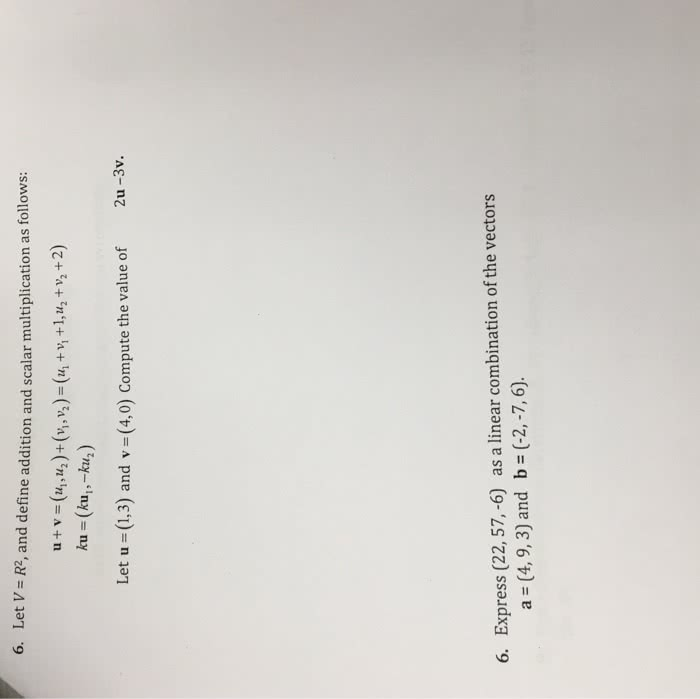1
0
watching
18
views13 Nov 20196. L et V = R2, and define addition and scalar multiplication as follows: Let u (1,3) and v (4,0) Compute the value of 2u -3v. 6. Express (22, 57,-6) as a linear combination of the vectors b = (-2,-7,6). a = 14, 93) and